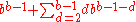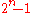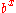xPandigital numberEncyclopedia
In mathematics
Mathematics
Mathematics is the study of quantity, space, structure, and change. Mathematicians seek out patterns and formulate new conjectures. Mathematicians resolve the truth or falsity of conjectures by mathematical proofs, which are arguments sufficient to convince other mathematicians of their validity...

, a pandigital number is an integer that in a given base has among its significant digits each digit used in the base at least once. For example, 1223334444555567890 is a pandigital number in base 10. The first few pandigital base 10 numbers are given by :

1023456789, 1023456798, 1023456879, 1023456897, 1023456978, 1023456987, 1023457689

The smallest pandigital number in a given base b is an integer of the formThe following table lists the smallest pandigital numbers of a few selected bases:
Base Smallest pandigital Values in base 10
2 10 2
3 102 11
11 (number)
11 is the natural number following 10 and preceding 12.Eleven is the first number which cannot be counted with a human's eight fingers and two thumbs additively. In English, it is the smallest positive integer requiring three syllables and the largest prime number with a single-morpheme name...

4 1023 75
75 (number)
75 is the natural number following 74 and preceding 76.-In mathematics:As the sum of the first five pentagonal numbers, seventy-five is a pentagonal pyramidal number. It is also an enneagonal number....

10 1023456789 1023456789
16 1023456789ABCDEF 1162849439785405935
Roman
numerals
MCDXLIV 1444

gives the base 10 values for the first 18 bases.

In a trivial sense, all positive integers are pandigital in unary (or tallying). In binary, all integers are pandigital except for 0 and numbers of the form(the Mersenne number
Mersenne prime
In mathematics, a Mersenne number, named after Marin Mersenne , is a positive integer that is one less than a power of two: M_p=2^p-1.\,...

s). The larger the base, the rarer pandigital numbers become, though one can always find runs ofconsecutive pandigital numbers with redundant digits by writing all the digits of the base together (but not putting the zero first as the most significant digit) and adding x + 1 zeroes at the end as least significant digits.

Conversely, the smaller the base, the fewer pandigital numbers without redundant digits there are. 2 is the only such pandigital number in base 2, while there are more of these in base 10.

Sometimes, the term is used to refer only to pandigital numbers with no redundant digits. In some cases, a number might be called pandigital even if it doesn't have a zero as a significant digit, for example, 923456781 (these are sometimes referred to as "zeroless pandigital numbers").

No base 10 pandigital number can be a prime number
Prime number
A prime number is a natural number greater than 1 that has no positive divisors other than 1 and itself. A natural number greater than 1 that is not a prime number is called a composite number. For example 5 is prime, as only 1 and 5 divide it, whereas 6 is composite, since it has the divisors 2...

if it doesn't have redundant digits. The sum of the digits 0 to 9 is 45, passing the divisibility rule
Divisibility rule
A divisibility rule is a shorthand way of discovering whether a given number is divisible by a fixed divisor without performing the division, usually by examining its digits...

for both 3 and 9. The first base 10 pandigital prime is 10123457689; lists more.

For different reasons, redundant digits are also required for a pandigital number (in any base except unary) to also be a palindromic number
Palindromic number
A palindromic number or numeral palindrome is a 'symmetrical' number like 16461, that remains the same when its digits are reversed. The term palindromic is derived from palindrome, which refers to a word like rotor that remains unchanged under reversal of its letters...

in that base. The smallest pandigital palindromic number in base 10 is 1023456789876543201.

The largest pandigital number without redundant digits to be also a square number
Square number
In mathematics, a square number, sometimes also called a perfect square, is an integer that is the square of an integer; in other words, it is the product of some integer with itself...

is 9814072356
9814072356 (number)
9814072356 or 9,814,072,356 is 99066 squared, and is the eighty-seventh and largest square number using each of the digits 1, 2, 3, 4, 5, 6, 7, 8, 9, and 0 exactly once ....

.

Two of the zeroless pandigital Friedman number
Friedman number
A Friedman number is an integer which, in a given base, is the result of an expression using all its own digits in combination with any of the four basic arithmetic operators and sometimes exponentiation. For example, 347 is a Friedman number since 347 = 73 + 4...

s are: 123456789 = ((86 + 2 × 7)5 - 91) / 34, and 987654321 = (8 × (97 + 6/2)5 + 1) / 34.

While much of what has been said does not apply to Roman numerals, there are pandigital numbers: MCDXLIV, MCDXLVI, MCDLXIV, MCDLXVI, MDCXLIV, MDCXLVI, MDCLXIV, MDCLXVI. These, listed in , use each of the digits just once, while has pandigital Roman numerals with repeats.

Pandigital numbers are useful in fiction and in advertising. The Social Security Number
Social Security number
In the United States, a Social Security number is a nine-digit number issued to U.S. citizens, permanent residents, and temporary residents under section 205 of the Social Security Act, codified as . The number is issued to an individual by the Social Security Administration, an independent...

987-65-4321 is a zeroless pandigital number reserved for use in advertising. Some credit card companies use pandigital numbers with redundant digits as fictitious credit card numbers (while others use strings of zeroes).

## Examples of pandigital numbers

• 123456789 = The first zeroless pandigital number.
• 987654321 = The largest zeroless pandigital number without redundant digits.
• 1023456789 = The first pandigital number.
• 1234567890 = The first pandigital number with the digits in order.
• 9876543210 = The largest pandigital number without redundant digits.
• 12345678987654321 = A pandigital number with all the digits except zero in both ascending and descending order. It is the square of 111111111. It is also a palindrome number.